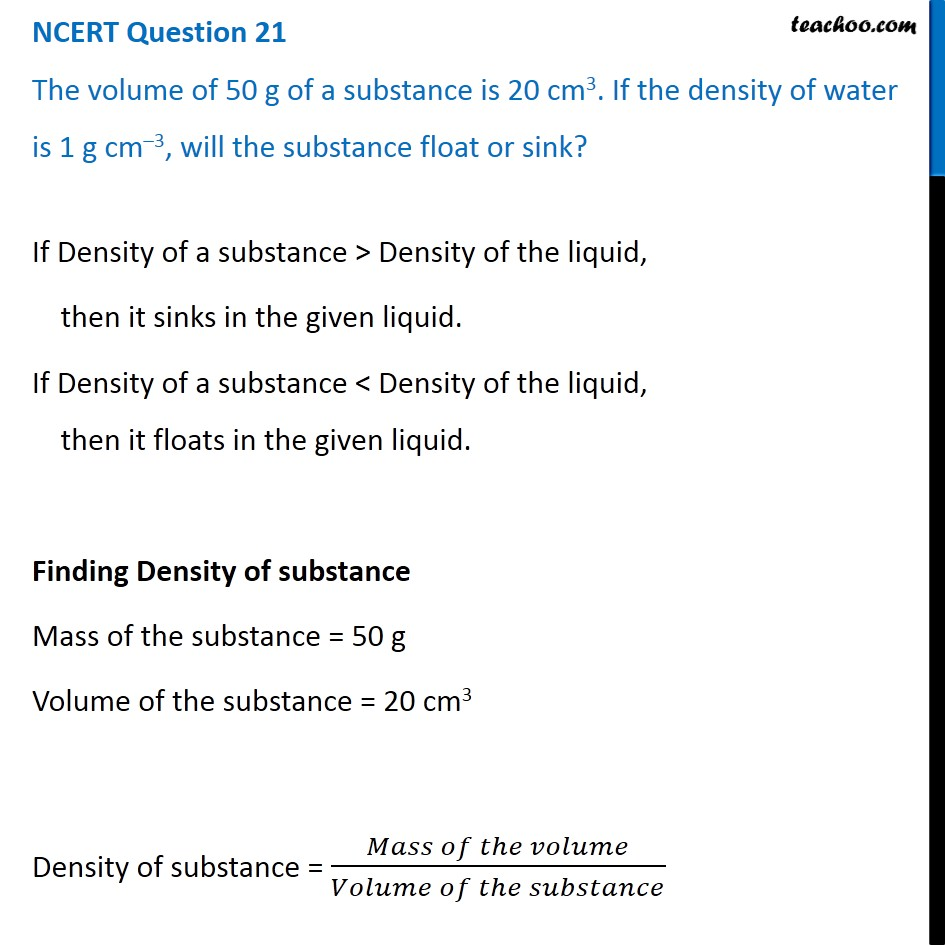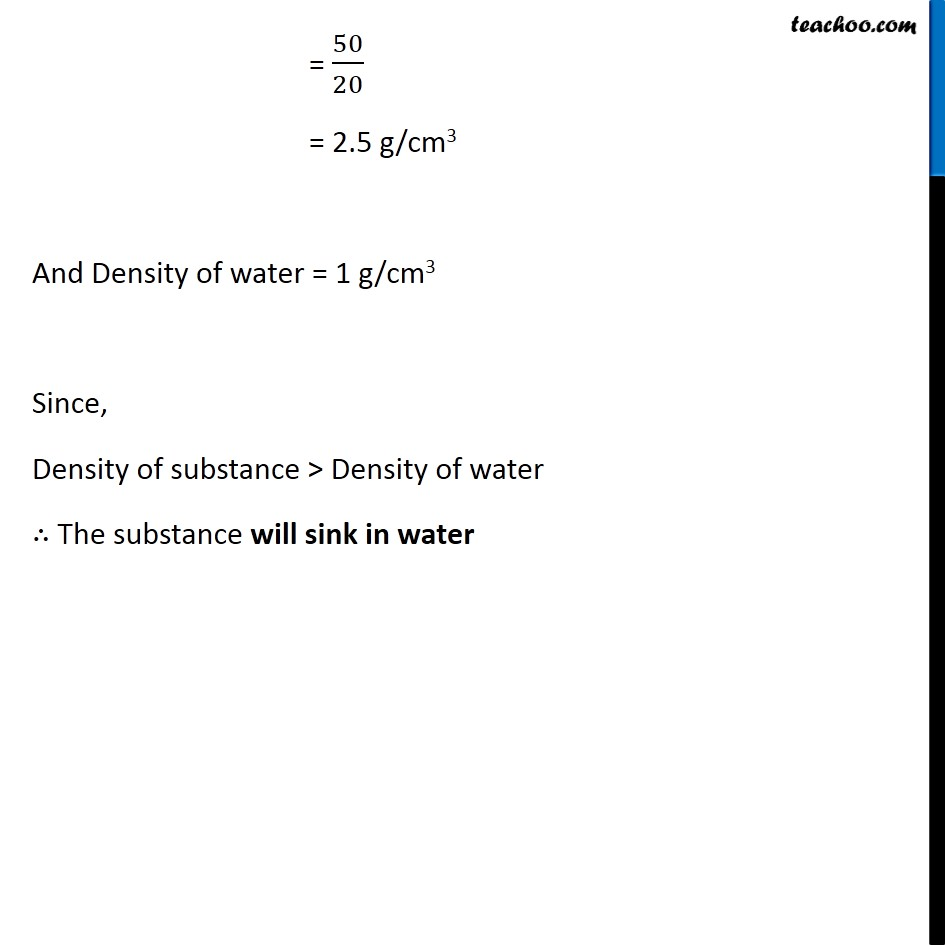NCERT Questions

Class 9
Chapter 10 Class 9 - GravitationLearn in your speed, with individual attention - Teachoo Maths 1-on-1 Class

### Transcript

NCERT Question 21 The volume of 50 g of a substance is 20 cm3. If the density of water is 1 g cm–3, will the substance float or sink? If Density of a substance > Density of the liquid, then it sinks in the given liquid. If Density of a substance < Density of the liquid, then it floats in the given liquid. Finding Density of substance Mass of the substance = 50 g Volume of the substance = 20 cm3 Density of substance = (𝑀𝑎𝑠𝑠 𝑜𝑓 𝑡ℎ𝑒 𝑣𝑜𝑙𝑢𝑚𝑒)/(𝑉𝑜𝑙𝑢𝑚𝑒 𝑜𝑓 𝑡ℎ𝑒 𝑠𝑢𝑏𝑠𝑡𝑎𝑛𝑐𝑒) = 50/20 = 2.5 g/cm3 And Density of water = 1 g/cm3 Since, Density of substance > Density of water ∴ The substance will sink in water